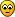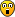## XY chain problem

Programs which generate, solve, and analyze Sudoku puzzles

### Re: XY chain problem

Hajime wrote:
yzfwsf wrote:(5 = 7) r3c2 and (7 = 6) r6c1 are not in the same house, and the candidates(5 start/6 end) are not the same, so you cannot form any eliminations.

Can XYchain not be reversed? So head/tail swapped? Than (7start/7 end)

No no, not so bright Hajime ! The 5 and 6 needs still to be compared in a reverse XYchain. So no valid XYchain.

Thank you yzfwsf. I am convinced that all XYchains can be reversed.
So to find an XYchain for a certain cell you only have to search for two pincer cells (head/tail) where the head-cell is earlier (in reading order) than the tail cell.

Next question:
I like the two-coloring of candidates of the images. So if we search for a 5 in the head (cell 1) and tail (cell n), that must be of different colors (say orange/green) to have a valid XYchain. Let 5 in head-cell be orange. Than a green link will be there from cell 1 to cell 2, an orange link between cell 2 and cell 3, etc. We need to end with an orange link between cell n-1 and cell n. Conclusion: the length of an XYchain has always an odd number. Right?
Do your solvers stop searching after the first found XYchain or do you proceed to find a possible shorter chain?
Is there an XYchain in the above puzzle of length 5? You only have to find one elimination in this puzzle to have stte.Hajime

Posts: 1270
Joined: 20 April 2018
Location: Fryslân

### Re: XY chain problem

Hajime wrote: I am convinced that all XYchains can be reversed.

Yes, your idea is very correct. Not only XY chain can be reversed, but also the more common AIC can be reversed. The starting point and end point of AIC are strong relationships, which determines that it can be reversed. XY chain is only a special case of AIC, and all strong relations exist in the same cell.

Hajime wrote: Do your solvers stop searching after the first found XYchain or do you proceed to find a possible shorter chain?

Yes,my solver will stop searching after the first found AIC(mybe it is XY-Chain).

Hajime wrote: Is there an XYchain in the above puzzle of length 5? You only have to find one elimination in this puzzle to have stte.

My solver doesn't consider chain length as a sort parameter. It has a function of searching all possible techniques in different PM States, but in the new version, only one such chain will be presented, because they are completely equivalent.
yzfwsf

Posts: 716
Joined: 16 April 2019

### Re: XY chain problem

Hajime wrote:Conclusion: the length of an XYchain has always an odd number. Right?

Wrong?: (5=7)r3c2 (7=9)r2c1 (9=1)r2c7 (1=7)r2c9 (7=6)r1c8 (6=7)r6c8 (7=6)r6c1 (6=5)r7c1 => (-5)r5c7Hajime

Posts: 1270
Joined: 20 April 2018
Location: Fryslân

### Re: XY chain problem

I think these are all the possible XYchains
Code: Select all
`XY_Chain      (1=9)r2c7 (9=7)r2c1 (7=5)r3c2 (5=6)r7c2 (6=7)r5c2 (7=6)r6c1 (6=7)r6c8 (7=3)r5c9 (3=1)r8c9>> (-1)r2c9 or r8c7    (3=7)r5c9 (7=6)r5c2 (6=7)r6c1 (7=9)r2c1 (9=1)r2c7 (1=3)r8c7>> (-3)r5c7 or r8c9    (5=7)r3c2 (7=9)r2c1 (9=1)r2c7 (1=7)r2c9 (7=6)r1c8 (6=7)r6c8 (7=6)r6c1 (6=5)r7c1>> (-5)r3c1 or r7c2     (6=7)r1c8 (7=9)r1c5 (9=7)r3c5 (7=5)r3c2 (5=6)r7c2 (6=7)r5c2 (7=6)r6c1 (6=7)r6c8 (7=3)r5c9 (3=6)r5c7>> (-6)r1c7 or r5c8    (6=7)r1c8 (7=9)r1c5 (9=7)r3c5 (7=5)r3c2 (5=6)r7c2 (6=7)r5c2 (7=6)r6c1>> (-6)r1c1 or r6c8    (6=3)r5c7 (3=7)r5c9 (7=1)r2c9 (1=9)r2c7 (9=7)r2c1 (7=5)r3c2 (5=6)r7c2>> (-6)r5c2 or r7c7   (6=7)r6c1 (7=9)r2c1 (9=1)r2c7 (1=7)r2c9 (7=6)r1c8 (6=7)r6c8 (7=3)r5c9 (3=6)r5c7 (6=7)r5c2 (7=5)r3c2 (5=6)r7c2>> (-6)r6c2 or r7c1    (7=9)r1c5 (9=6)r1c7 (6=7)r1c8 (7=1)r2c9 (1=3)r8c9 (3=7)r5c9 (7=6)r5c2 (6=5)r7c2 (5=7)r3c2>> (-7)r1c2 or r3c5    (7=9)r1c5 (9=7)r3c5 (7=5)r3c2 (5=6)r7c2 (6=7)r5c2 (7=6)r6c1 (6=7)r6c8>> (-7)r1c8 or r6c5    (7=9)r2c1 (9=1)r2c7 (1=7)r2c9 (7=6)r1c8 (6=7)r6c8>> (-7)r2c8 or r6c1    (7=1)r2c9 (1=9)r2c7 (9=7)r2c1 (7=5)r3c2 (5=6)r7c2 (6=7)r5c2>> (-7)r2c2 or r5c9    (7=1)r2c9 (1=9)r2c7 (9=6)r1c7 (6=7)r1c8 (7=6)r6c8 (6=7)r6c1>> (-7)r2c1 or r6c9    (7=9)r3c5 (9=7)r1c5 (7=6)r1c8 (6=9)r1c7 (9=1)r2c7 (1=7)r2c9 (7=3)r5c9 (3=6)r5c7 (6=7)r5c2>> (-7)r3c2 or r5c5   (9=6)r1c7 (6=7)r1c8 (7=9)r1c5 (9=7)r3c5 (7=5)r3c2 (5=6)r7c2 (6=7)r5c2 (7=6)r6c1 (6=7)r6c8 (7=3)r5c9 (3=1)r8c9 (1=7)r2c9 (7=9)r2c1>> (-9)r1c1 or r2c7    (9=6)r1c7 (6=7)r1c8 (7=1)r2c9 (1=3)r8c9 (3=7)r5c9 (7=6)r5c2 (6=5)r7c2 (5=7)r3c2 (7=9)r3c5>> (-9)r1c5 or r3c7   (9=7)r2c1 (7=5)r3c2 (5=6)r7c2 (6=7)r5c2 (7=6)r6c1 (6=7)r6c8 (7=6)r1c8 (6=9)r1c7 (9=7)r1c5 (7=9)r3c5>> (-9)r2c5 or r3c1`

but it takes 15 seconds to find them all. And after some candidates are eliminated, normally other XYchains are not valid anymore.
There is one XYchain of length 5 though... and if I stop after the first of length 9, I am not that happyHmmm nedBlake 's XYchain is not included....Hajime

Posts: 1270
Joined: 20 April 2018
Location: Fryslân

### Re: XY chain problem

I can see that you now correctly show the inferences order within the cell as you read the nodes from left to right. This would then show you that you can read the same chain from right to left.

Ned's is a special case where the start node & end node share a sector (column in this instance). The assertion that r1c2 = 7 will lead (if you follow the chain) into r5c2 = 7 which can't happen therefore the assumption r1c2 = 7 must be false.

Like X chains you can use colouring (multi colouring in this instance) to make visualizing it easiertarek

Posts: 3761
Joined: 05 January 2006

Previous